Your data analysis solution

# Sample size for a clinical trial tutorial in Excel

2017-10-20

This tutorial will help you compute sample size for a clinical trial in Excel using the XLSTAT statistical software.

## Goal of this tutorial

This tutorial aims at showing how to estimate the necessary sample size in classical clinical trials.

When organizing a trial, the first question that arises is the number of patient to be included in order to obtain statistically valid results.

Three types of trials can be studied:

• Equivalence trials: An equivalence trial is where you want to demonstrate that a new treatment is no better or worse than an existing treatment.
• Superiority trials: A superiority trial is one where you want to demonstrate that one treatment is better than another.
• Non-inferiority trials: A non-inferiority trial is one where you want to show that a new treatment is not worse than an existing treatment.

These tests can be applied to a binary outcome or a continuous outcome.

We will give three examples for each one of these trials. You can find the results

## Dataset for the calculation of the necessary sample size

An Excel spreadsheet containing the results of this example can be downloaded by clicking here.

## Setting up of the calculation of the necessary sample size for a clinical trial

After opening XLSTAT, click the Power icon and choose clinical trials.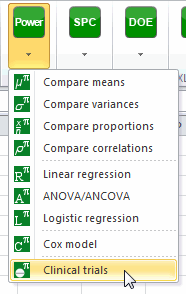Once the button is clicked, the dialog box appears.

## Superiority trial

A new treatment has been obtained, its expected results on patients should be better than the actual treatment. We wish to know the sample size necessary to reject the null hypothesis that both treatments have the same effect.

The outcome is the fact that a patient is cured or not. It is a binary outcome. We suppose that the control group (with the oldest treatment) has a recovery of 60% and the treatment group (with the new treatment) has a recovery of 70%. We wish to estimate necessary sample size for a level of 5% and a power of 0.9.

You must then choose the objective Find the sample size, then select the superiority trial and the binary outcome. The alpha is 0.05. The desired power is 0.9. The % of success for the control group is 60 and the % of success for the treatment group is 70. There is no cross-over.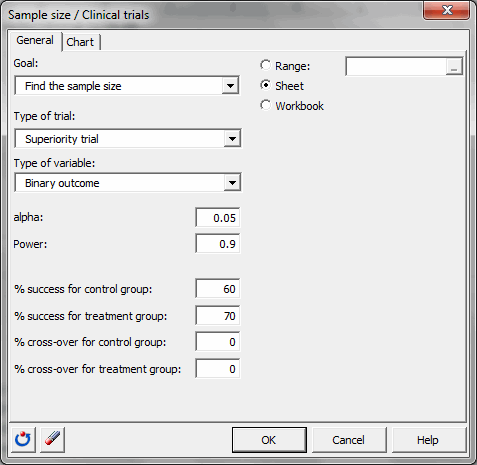Once you have clicked the OK button, the calculations are made, and then the results are displayed.

#### Results of the calculation of the necessary sample size for a superiority trial

The first table shows the calculation results and an interpretation of the results.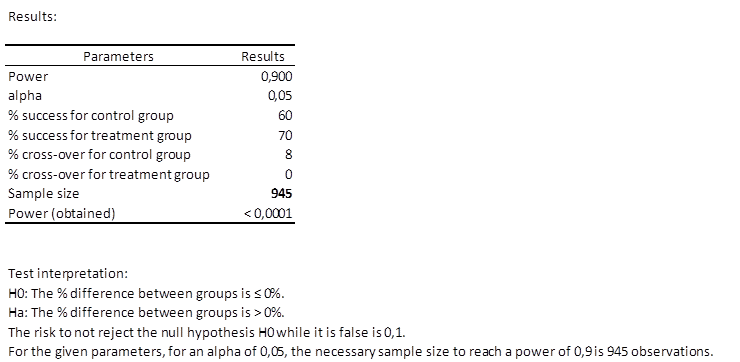We can see that 945 patients are necessary, which means two groups of at least 473 patients.

The simulation plot gives you more information on the evolution of the sample size with respect to power.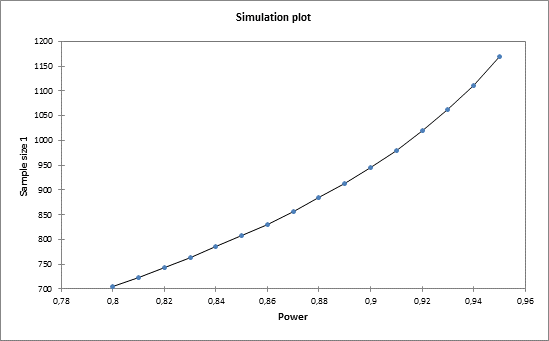## Equivalence trial

A new treatment has been obtained, it has way less side effects than the classical treatment. We wish to be sure that both treatments are equivalent. To do so, we wish to test that both treatment are in the same range of results.

We use equivalence testing. An equivalence limit should be defined. In our case we define an equivalence limit of 10%.

The outcome is the fact that a patient is cured or not. It is a binary outcome. We suppose that the control group (with the oldest treatment) and the treatment group (with the new treatment) have a recovery of 60%. We wish to estimate necessary sample size for a level of 5% and a power of 0.9.

You must then choose the objective Find the sample size, then select the superiority trial and the binary outcome. The alpha is 0.05. The desired power is 0.9. The % of success for both groups is 60 and the equivalence limit is equal to 10.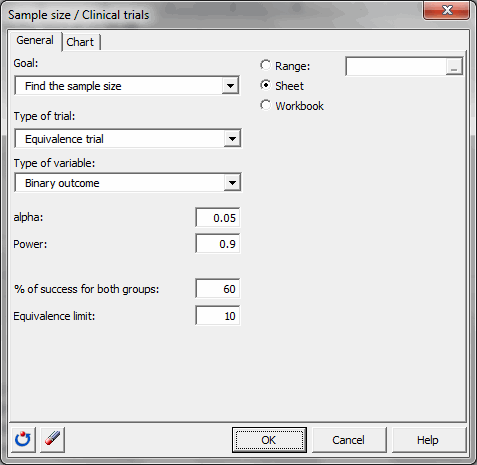Once you have clicked the OK button, the calculations are made, and then the results are displayed.

#### Results of the calculation of the necessary sample size for an equivalence trial

The first table shows the calculation results and an interpretation of the results.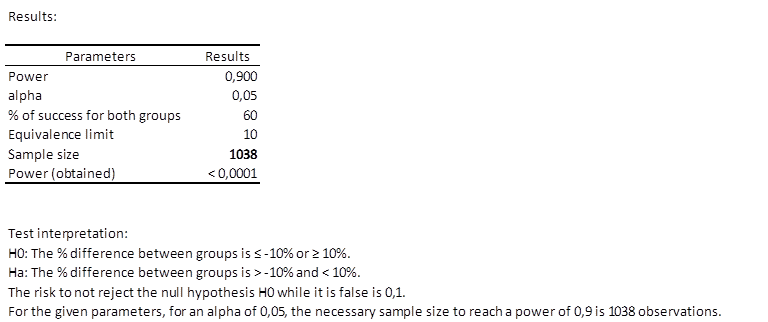We can see that 1038 patients are necessary, which means two groups of at least 519 patients.

The simulation plot gives you more information on the evolution of the sample size with respect to power.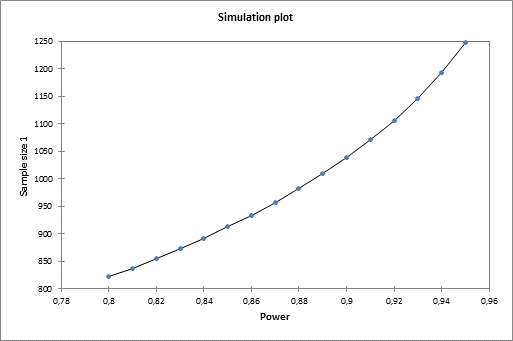## Non-inferiority trial

A new treatment has been obtained, it is cheaper than the actual treatment. We wish to be sure that the new treatment has an at least equal or a little bit less effective effect than the actual treatment. To do so, we wish to test that the new treatment is better or slightly worst than the original treatment.

We use non-inferiority testing. A non-inferiority limit should be defined. In our case we define a non-inferiority limit of 8%.

The outcome is the fact that a patient is cured or not. It is a binary outcome. We suppose that the control group (with the oldest treatment) has a recovery of 70% and the treatment group (with the new treatment) has a recovery of 75%. We wish to estimate necessary sample size for a level of 5% and a power of 0.9.

You must then choose the objective Find the sample size, then select the non-inferiority trial and the binary outcome. The alpha is 0.05. The desired power is 0.9. The % of success for the control group is 70 and the % of success for the treatment group is 75. The non-inferiority limit is equal to 8..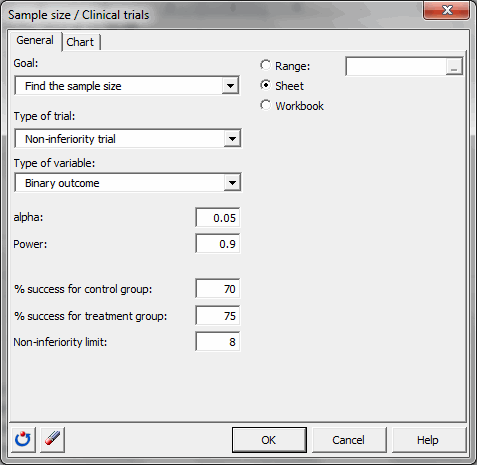Once you have clicked the OK button, the calculations are made, and then the results are displayed.

#### Results of the calculation of the necessary sample size for a non-inferiority trial

The first table shows the calculation results and an interpretation of the results.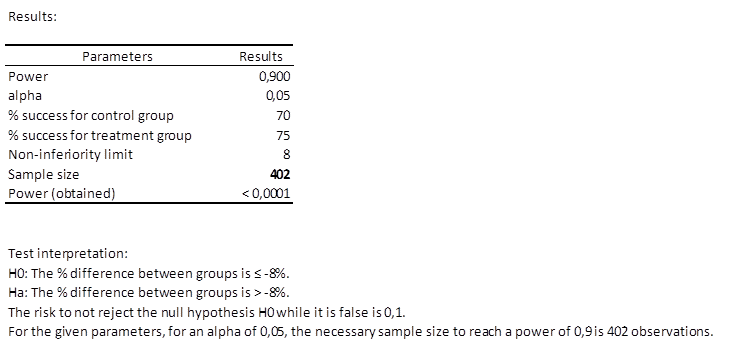We can see that only 402 patients are necessary, which means two groups of at least 201 patients.

The simulation plot gives you more information on the evolution of the sample size with respect to power.## Conclusions

XLSTAT gives you the opportunity to obtain necessary sample size for many kind of trials. We have seen that non-inferiority trials are less restrictive than the others but are also more controversial.

XSLTAT enables you to find the power and necessary sample size when the outcome variable is continuous and to represent many simulation plots.

### Contact Us

#### Contact our technical support team: support@xlstat.com

https://cdn.desk.com/
false
desk
Loading
seconds ago
a minute ago
minutes ago
an hour ago
hours ago
a day ago
days ago
about
false
Invalid characters found
/customer/portal/articles/autocomplete
9283